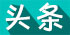栏目ID=19的表不存在(操作类型=0)

栏目ID=19的表不存在(操作类型=0)

栏目ID=19的表不存在(操作类型=0)

栏目ID=19的表不存在(操作类型=0)

栏目ID=25的表不存在(操作类型=0)
栏目ID=25的表不存在(操作类型=0)

栏目ID=29的表不存在(操作类型=0)
栏目ID=28的表不存在(操作类型=0)
栏目ID=27的表不存在(操作类型=0)
栏目ID=26的表不存在(操作类型=0)

栏目ID=21的表不存在(操作类型=0)

栏目ID=29的表不存在(操作类型=0)
栏目ID=30的表不存在(操作类型=0)
栏目ID=20的表不存在(操作类型=0)
栏目ID=22的表不存在(操作类型=0)
栏目ID=23的表不存在(操作类型=0)